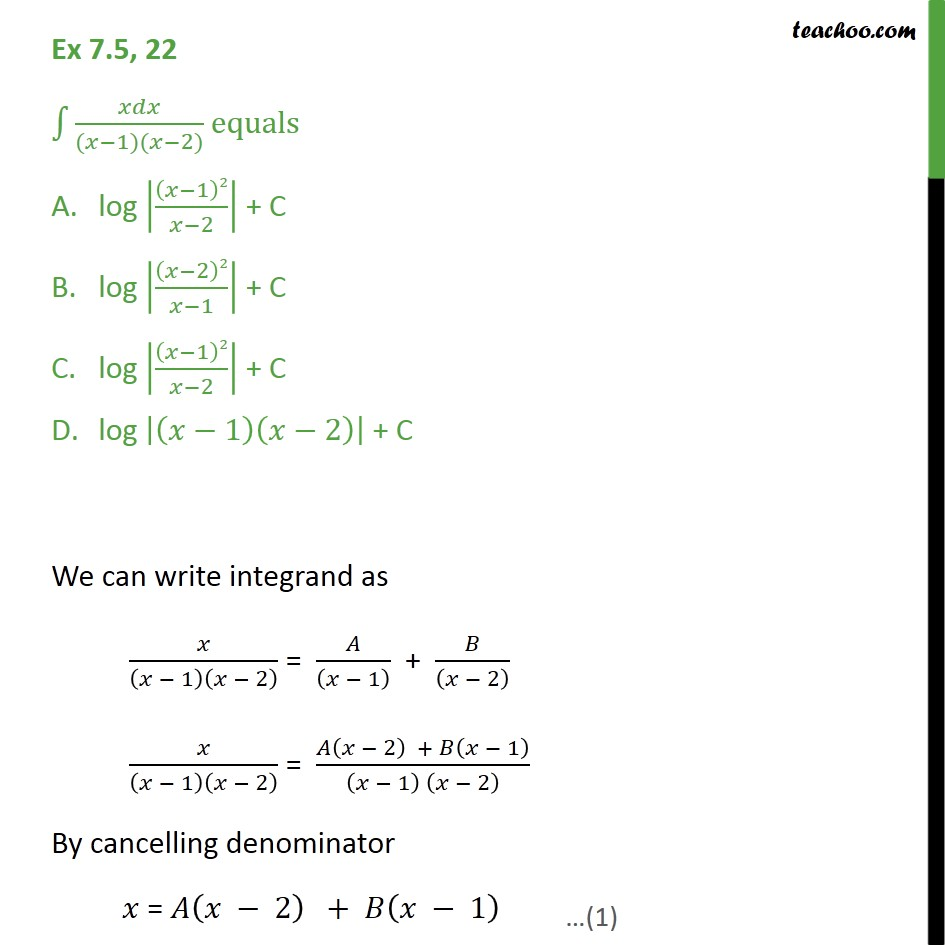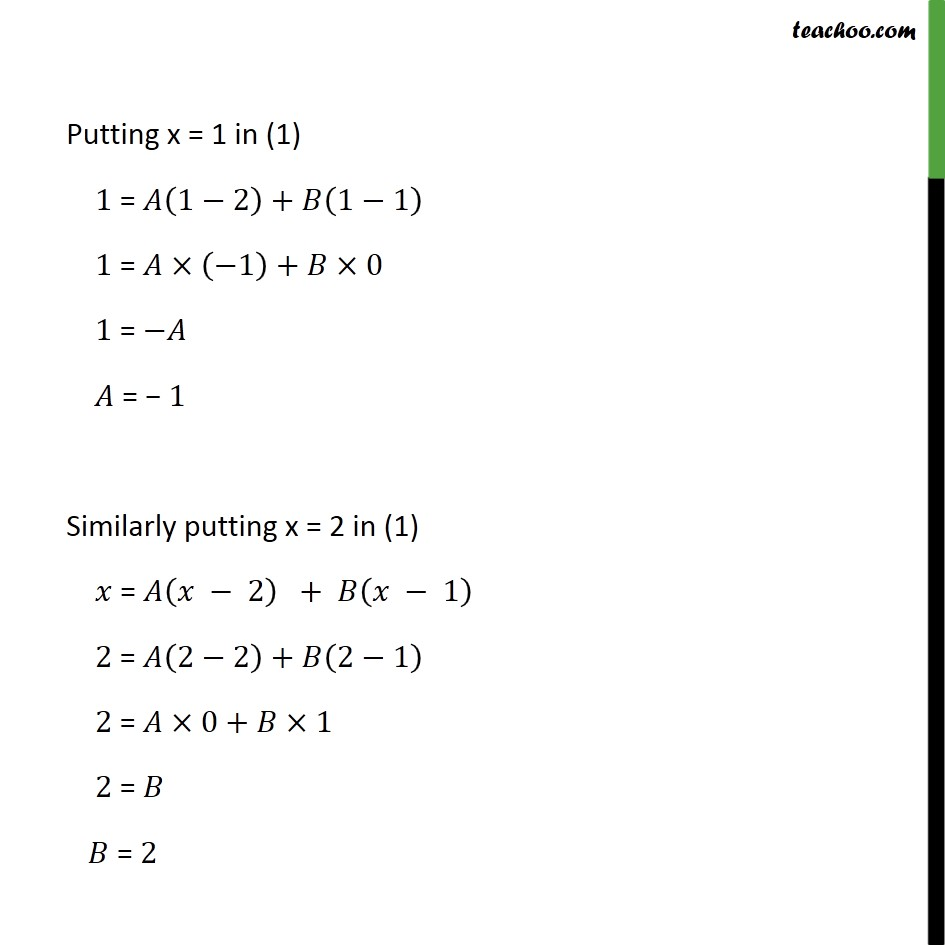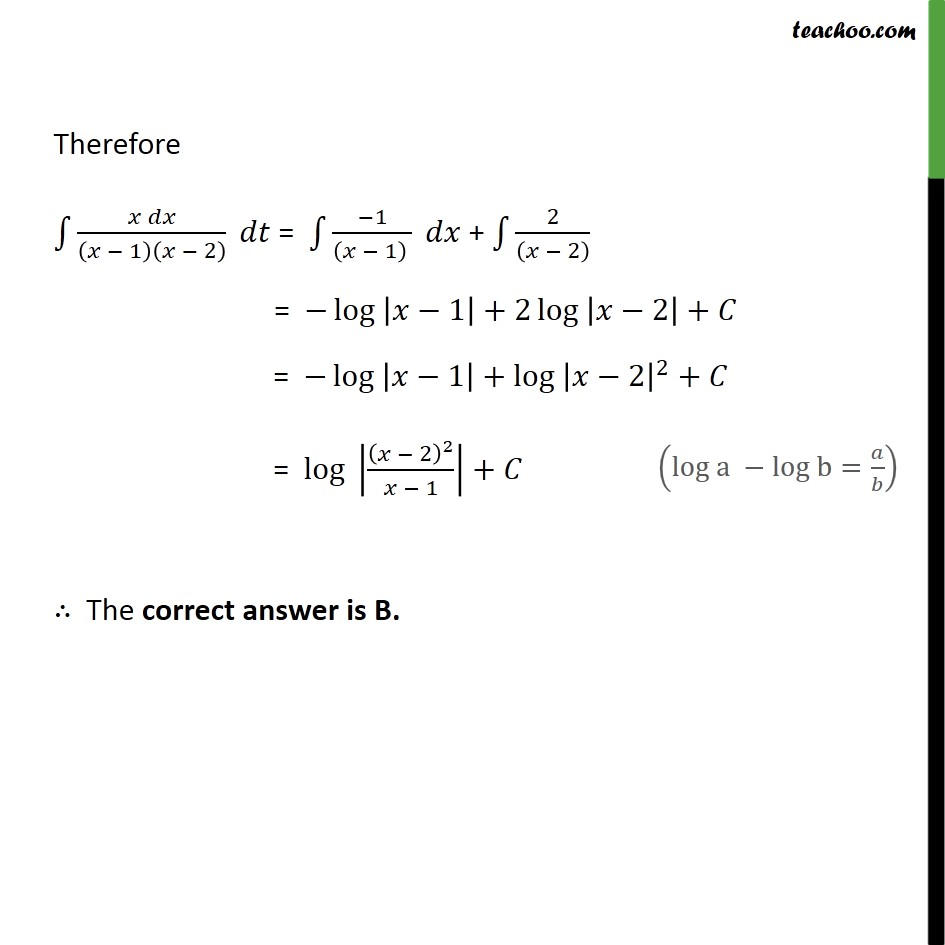Ex 7.5

Chapter 7 Class 12 Integrals
Serial order wiseLearn in your speed, with individual attention - Teachoo Maths 1-on-1 Class

### Transcript

Ex 7.5, 22 ﷮﷮ 𝑥𝑑𝑥﷮(𝑥−1)(𝑥−2)﷯﷯ equals • log 𝑥−1﷯2﷮𝑥−2﷯﷯ + C • log 𝑥−2﷯2﷮𝑥−1﷯﷯ + C • log 𝑥−1﷯2﷮𝑥−2﷯﷯ + C • log 𝑥−1﷯ 𝑥−2﷯﷯ + C We can write integrand as 𝑥﷮ 𝑥 − 1﷯ 𝑥 − 2﷯﷯ = 𝐴﷮ 𝑥 − 1﷯﷯ + 𝐵﷮ 𝑥 − 2﷯﷯ 𝑥﷮ 𝑥 − 1﷯ 𝑥 − 2﷯﷯ = 𝐴 𝑥 − 2﷯ + 𝐵 𝑥 − 1﷯﷮ 𝑥 − 1﷯ 𝑥 − 2﷯﷯ By cancelling denominator 𝑥 = 𝐴 𝑥 − 2﷯ + 𝐵 𝑥 − 1﷯ Putting x = 1 in (1) 1 = 𝐴 1−2﷯+𝐵 1−1﷯ 1 = 𝐴× −1﷯+𝐵×0 1 = −𝐴 𝐴 = − 1 Similarly putting x = 2 in (1) 𝑥 = 𝐴 𝑥 − 2﷯ + 𝐵 𝑥 − 1﷯ 2 = 𝐴 2−2﷯+𝐵 2−1﷯ 2 = 𝐴×0+𝐵×1 2 = 𝐵 𝐵 = 2 Therefore ﷮﷮ 𝑥 𝑑𝑥﷮ 𝑥 − 1﷯ 𝑥 − 2﷯﷯﷯ 𝑑𝑡 = ﷮﷮ −1﷮ 𝑥 − 1﷯ ﷯﷯ 𝑑𝑥 + ﷮﷮ 2﷮ 𝑥 − 2﷯﷯﷯ = − log ﷮ 𝑥−1﷯﷯+2 log ﷮ 𝑥−2﷯﷯+𝐶 = − log ﷮ 𝑥−1﷯﷯+ log ﷮ 𝑥−2﷯﷮2﷯﷯+𝐶 = log ﷮ 𝑥 − 2﷯﷮2﷯﷮𝑥 − 1﷯﷯﷯+𝐶 ∴ The correct answer is B.